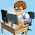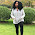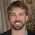Solutions to Introduction to Java Programming ExercisesBelow are links to the solutions to Y. Daniel Liang's Introduction To Java Programming (9th Edition) exercises that have been posted so far. This page is updated as more solutions to the exercises are posted. You are "STRONGLY ADVISED" to try to solve the problem first before checking the solution. Note that there are multiple ways of solving problems. That is to say, you may have another way (maybe even better) of solving the problem(s). In such case(s) please let us know. Only solutions to programming exercises are provided here. You can test run the programs online for free at ideone.com.

Chapter One

Exercise 1.1 - Java program to display three messages
Exercise 1.2 - Java program to display five messages
Exercise 1.3 - Java program to display pattern
Exercise 1.4 - Java program to print table
Exercise 1.5 - Computing mathematical expressions in Java
Exercise 1.6 - Summation of a series
Exercise 1.7 - Program to calculate approximate value of pi
Exercise 1.8 - Program to calculate area and perimeter of a circle
Exercise 1.9 - Java program to calculate area and perimeter of a rectangle
Exercise 1.10 - Java program to calculate average speed in miles
Exercise 1.11 - Population projection
Exercise 1.12 - Java program to calculate average speed in kilometers

Chapter Two

Exercise 2.1 - Program to convert celsius to fahrenheit
Exercise 2.2 - Volume of a cylinder calculator in Java
Exercise 2.3 - Feet to meters calculator in Java
Exercise 2.4 - Pounds to kilograms calculator
Exercise 2.5 - Financial application
Exercise 2.6 - Java program to sum the digits in an integer
Exercise 2.7 - Program to find number of years
Exercise 2.8 - Java program to display current time in GMT
Exercise 2.9 - Java physics program to calculate average acceleration
Exercise 2.10 - Java program to calculate energy
Exercise 2.11 - Population projection
Exercise 2.12 - Physics: finding runway length
Exercise 2.13 - Financial application: compound value
Exercise 2.14 - Health application: computing BMI
Exercise 2.16 - Geometry: area of a hexagon
Exercise 2.20 - Financial application: calculate interest
Exercise 2.21 - Financial application: calculate future investment value
Exercise 2.22 - Random character
Exercise 2.23 - Find the character of an ASCII code
Exercise 2.24 - Financial application: monetary units
Exercise 2.25 - Financial application: payroll
Exercise 2.26 - Use input dialog
Exercise 2.27 - Financial application: payroll

Chapter Three

Exercise 3.1 - Algebra: solve quadratic equations
Exercise 3.2 - Game: add three numbers
Exercise 3.3 -  Algebra: Solve 2x2 Linear Equations
Exercise 3.4 - Game: Learn Addition
Exercise 3.5 - Find Future Dates
Exercise 3.6 - Health Application: BMI
Exercise 3.9 - Business: Check ISBN-10
Exercise 3.10 - Game: Addition Quiz
Exercise 3.11 - Find the Number of Days in a Month
Exercise 3.12 - Check a Number

1.where is the completed other chapters ? 2 3 4 ..etc

1.Check back again soon. I will be updating this page with each chapter as soon as I finish them. Meanwhile bookmark this page.

2.yo you finish the other chapters??????

3.No, not yet. Been very busy. I'll do that asap.

4.2.I still wait to completed this book

1.Hello how are you this is very useful to me I learn java on my own thank you so was wondering are you going to upload the other chapters or were can I find them

3.Do You finish the other chapters?

1.No still working on it. Will upload them soon.

4.1.I'm glad you find it useful. I'm still working on it. Just check back again. Subscribe to the email newsletter (at the top right) to be notified immediately it is uploaded.

2.5.Thank you Aghatise Osazuwa for your efforts. I'm doing a similar work but for the 10th edition of this book. My soulutins can be found on github.

1.Thanks but where are the others chapter ? Ed 10 right ? It seems like the author in that book still mention Ed 9 for chapter 33 to 50

Did you tackle them or not, ? please update

6.Hello! I just wanted to know by any chance if you happen to have the solution for exercise 13.25? Because the link you provided only has exerise 13.01-13.21

7.Solutions here are more advanced than explained in the book to chapter 3. (Sorry for my english)

8.If you go to the book's website at http://www.cs.armstrong.edu/liang/intro9e/exercisesolution.html you can find the solutions to all the even number problems.

9.Hi, can anyone tell me if there is a paperback version of this book? if there is please simply send me the ISBN number to the following email (darryoosh2012@gmail.com)